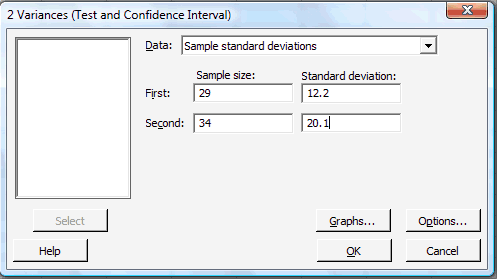# 12.3 - Using Minitab

12.3 - Using Minitab

In each case, we'll illustrate how to perform the hypothesis tests of this lesson using summarized data.

## Hypothesis Test for One Variance

1. Under the Stat menu, select Basic Statistics, and then select 1 Variance...:2. In the pop-up window that appears, in the box labeled Data, select Sample standard deviation (or alternatively Sample variance). In the box labeled Sample size, type in the size n of the sample. In the box labeled Sample standard deviation, type in the sample standard deviation. Click on the box labeled Perform hypothesis test, and in the box labeled Value, type in the Hypothesized standard deviation (or alternatively the Hypothesized variance):3. Click on the button labeled Options... In the pop-up window that appears, for the box labeled Alternative, select either less than, greater than, or not equal depending on the direction of the alternative hypothesis:4. Then, upon clicking OK on the main pop-up window, the output should appear in the Session window:

95% Confidence Intervals
Method CI for
StDev
CI for
Variance
Chi-Square (39.7,  62.3) (1578,  3878)
Tests
Method Test
Statistic
DF P-Value
Chi-Square 57.34 39 0.059

## Hypothesis Test for Two Variances

1. Under the Stat menu, select Basic Statistics, and then select 2 Variances...:2. In the pop-up window that appears, in the box labeled Data, select Sample standard deviations (or alternatively Sample variances). In the box labeled Sample size, type in the size n of the First sample and m of the Second sample. In the box labeled Standard deviation, type in the sample standard deviations for the First and Second samples:3. Click on the button labeled Options... In the pop-up window that appears, in the box labeled Value, type in the Hypothesized ratio of the standard deviations (or the Hypothesized ratio of the variances). For the box labeled Alternative, select either less than, greater than, or not equal depending on the direction of the alternative hypothesis:1. Then, upon clicking OK on the main pop-up window, the output should appear in the Session window:

### Test and CI for Two Variances

Method

Null hypothesis                 Sigma(1)  /  Sigma(2)  = 1
Alternative hypothesis     Sigma(1)  /  Sigma(2)  not  = 1
Significance level              Alpha  =  0.05

Statistics

Sample N StDev Variance
1 29 12.200 148.840
2 34 20.100 404.010

Ratio of standard deviations  =  0.607
Ratio of variances  =  0.368

95% Confidence Intervals

Distribution
of Data
CI for StDev Ratio CI for
Variance Ratio
Normal (0.425,  0.877) (0.180,  0.770)
Tests
Method DF1 DF2 Test
Statistic
P-Value
F Test (normal) 28 33 0.37 0.009

  Link ↥ Has Tooltip/Popover Toggleable Visibility